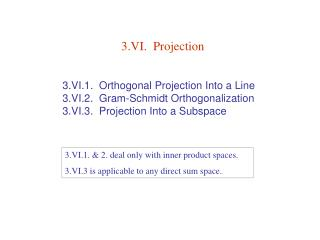# 3.VI. Projection - PowerPoint PPT PresentationDownload Presentation3.VI. Projection

3.VI. ProjectionDownload Presentation## 3.VI. Projection

- - - - - - - - - - - - - - - - - - - - - - - - - - - E N D - - - - - - - - - - - - - - - - - - - - - - - - - - -
##### Presentation Transcript

1. 3.VI. Projection 3.VI.1. Orthogonal Projection Into a Line 3.VI.2. Gram-Schmidt Orthogonalization 3.VI.3. Projection Into a Subspace 3.VI.1. & 2. deal only with inner product spaces. 3.VI.3 is applicable to any direct sum space.

2. 3.VI.1. Orthogonal Projection Into a Line Definition 1.1: Orthogonal Projection The orthogonal projectionofvinto the line spanned by a nonzerosis the vector. Example 1.3: Orthogonal projection of the vector ( 2 3 )T into the line y = 2x.

3. Example 1.4: Orthogonal projection of a general vector in R3 into the y-axis Example 1.5: Project = Discard orthogonal components A railroad car left on an east-west track without its brake is pushed by a wind blowing toward the northeast at fifteen miles per hour; what speed will the car reach?

4. Example 1.6: Nearest Point A submarine is tracking a ship moving along the line y = 3x + 2. Torpedo range is one-half mile. Can the sub stay where it is, at the origin on the chart, or must it move to reach a place where the ship will pass within range? Ship’s path is parallel to vector Point p of closest approach is (Out of range)

5. Exercises 3.VI.1. 1. Consider the function mapping a plane to itself that takes a vector to its projection into the line y = x. (a) Produce a matrix that describes the function’s action. (b) Show also that this map can be obtained by first rotating everything in the plane π/4 radians clockwise, then projecting into the x-axis, and then rotating π/4 radians counterclockwise.

6. 3.VI.2. Gram-Schmidt Orthogonalization Given a vector s, any vector v in an inner product space can be decomposed as where Definition 2.1: Mutually Orthogonal Vectors Vectors v1, …, vk Rnare mutually orthogonalif vi· vj = 0  i  j Theorem 2.2: A set of mutually orthogonal non-zero vectors is linearly independent. Proof: → → cj = 0  j Corollary 2.3: A set of k mutually orthogonal nonzero vectors in V kis a basis for the space. Definition 2.5: Orthogonal Basis An orthogonal basisfor a vector space is a basis of mutually orthogonal vectors.

7. Example 2.6: Turn into an orthogonal basis for R3.

8. Theorem 2.7: Gram-Schmidt Orthogonalization If  β1 , …, βk  is a basis for a subspace of Rnthen the κjs calculated from the following scheme is an orthogonal basis for the same subspace. Proof: For m 2 , Let  β1 , …, βm  be mutually orthogonal, and Then QED

9. If each κj is furthermore normalized, the basis is called orthonormal. The Gram-Schmidt scheme simplifies to:

10. Exercises 3.VI.2. 1. Perform the Gram-Schmidt process on this basis for R3, 2. Show that the columns of an nn matrix form an orthonormal set if and only if the inverse of the matrix is its transpose. Produce such a matrix.

11. 3.VI.3. Projection Into a Subspace Definition 3.1: For any direct sum V = M  N and any v V such that v= m + n with m M and n N The projection ofvinto M along Nis defined as projM, N(v) = m • Reminder: • M & N need not be orthogonal. • There need not even be an inner product defined.

12. Example 3.2: The space M22 of 22 matrices is the direct sum of Task: Find projM , N (A), where Solution: Let the bases for M & N be → is a basis for M22 . ∴

13. Example 3.3: Both subscripts on projM , N (v) are significant. Consider with basis & and subspaces It’s straightforward to verify Task: Find projM , N (v) and projM , L (v) where Solution: For →

14. For → Note: BML is orthogonal but BMN is not.

15. Definition 3.4: Orthogonal Complement The orthogonal complement of a subspace M of Rnis M= { vRn | vis perpendicular to all vectors in M } ( read “M perp” ). The orthogonal projection projM(v) of a vector is its projection into M along M. Example 3.5: In R3, find the orthogonal complement of the plane Solution: Natural basis for P is ( parameter = z) →

16. Lemma 3.7: Let M be a subspace of Rn. Then Mis also a subspace and Rn = M M . Hence,  v Rn, v projM (v) is perpendicular to all vectors in M. Proof: Construct bases using G-S orthogonalization. Theorem 3.8: Let vbe a vector in Rnand let M be a subspace of Rn with basis  β1 , …, βk . If Ais the matrix whose columns are the β’s then projM (v) = c1β1 + …+ ckβk where the coefficients ciare the entries of the vector (ATA) ATv. That is, projM (v) = A (ATA)1ATv. Proof: where c is a column vector → → → By lemma 3.7,

17. Example 3.9: To orthogonally project into subspace From we get →

18. Exercises 3.VI.3. 1. Project v into M along N, where 2. Find M for

19. 3. Define a projection to be a linear transformation t : V → V with the property that repeating the projection does nothing more than does the projection alone: ( t  t )(v) = t (v) for all v V. (a) Show that for any such t there is a basis B=  β1 , …, βn  for V such that where r is the rank of t. (b) Conclude that every projection has a block partial-identity representation: